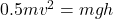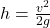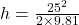## if a 0.4 kg baseball is thrown 25 mls straight into the air how high does it go

Question

if a 0.4 kg baseball is thrown 25 mls straight into the air how high does it go

in progress 0
3 weeks 2021-08-26T19:31:05+00:00 1 Answers 0 views 0

31.86

Explanation:

From the law of conservation of linear momentum, energy can neither be destroyed nor created but can only be transformed from one state to another. Kinetic energy in this case is converted to potential energyMaking h the subject of the formula thenHere m represent mass, g is acceleration due to gravity whose value is taken as 9.81, h is the height and v is the velocity. Substituting the given values thenh=31.86 m